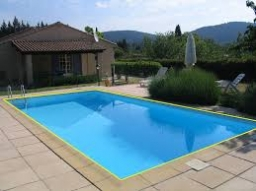# Hectoliters 48941

The pool has dimensions: a length of 50 m, a width of 15 m, and a depth of 2 m. It is filled with water to 1/4 of its depth. How many hectoliters of water are in the pool? How many hectoliters of water can fit in a filled pool?

V1 =  3750 hl
V =  15000 hl

### Step-by-step explanation:Did you find an error or inaccuracy? Feel free to write us. Thank you!

Tips for related online calculators
Need help calculating sum, simplifying, or multiplying fractions? Try our fraction calculator.
Do you know the volume and unit volume, and want to convert volume units?

#### Grade of the word problem:

We encourage you to watch this tutorial video on this math problem: